# 10 Math Applications for Android: Just Photo Problems!

Mathematics is known as a field of study that is hard to understand. There are a ton of difficult math problems found in the tests or quizzes and some of those who have to solve them are dizzy. Thanks to advanced technology, now answering math questions can be done on Android with applications. These applications are useful for answering, working on, and providing answers to difficult questions.

One of the things that make math hard is that there are many formulas that must be understood by students and society in general. Fortunately, the technology is growing and developers develop a variety of applications to solve math problems. For those who are looking for applications to solve math problems on Android, you might want to consider the following list recommended by Telset.id:

#### 1. MalMath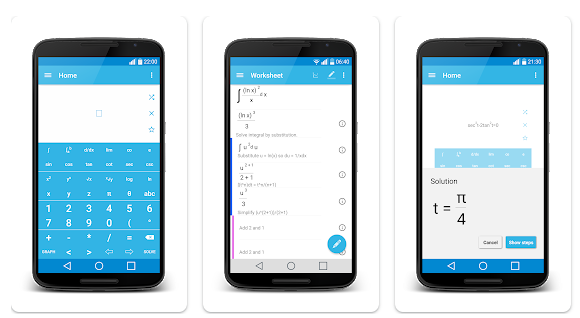If you have a hard time solving math problems, the best solution is to download MalMath. This one is available on Android and is perfect for students. This application has the ability to answer questions on integrals, derivatives, trigonometry, logarithms, and algebra. Aside from that, it is also equipped with a problem solving tutorial, making it easy for you to understand the process of how answers are found.

#### 2. PhotomathFor those who want to solve math quickly and practically, Photomath is perfect for you. In order to find answers to questions, all that you have to do is to take a photo of the question from the camera of your phone using this math answering application. After that, the application will do its job answering the questions quickly. Another good thing about this application is that it can be used offline and there are also several basic math topics in the language that can be understood easily.

#### 3. AutoMath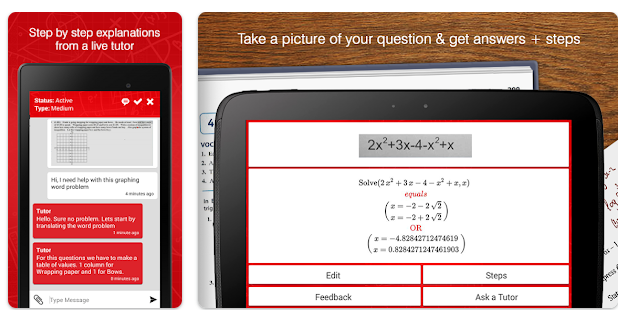Just like other applications for solving math problems, AutoMath is also able to provide answers to questions simply by taking a photo of the problem. Not only that, there are also more than 250 formulas that can be learned. With this application, you can solve a variety of math problems ranging from multiplication, division, roots of equations, algebra, to basic algorithms.

#### 4. MathWay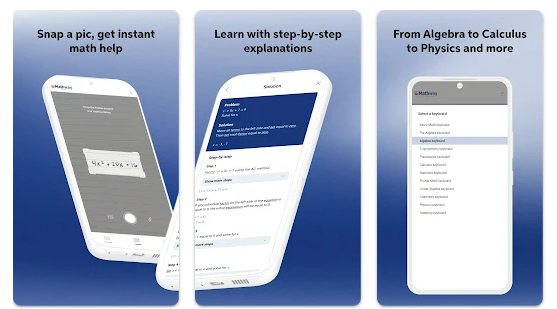For some people, answering math problems can be hard. If you also feel the same thing, then the solution is to download a math app such as MathWay. To use this application, you can just scan the questions in the application and the application will quickly answer them. Another thing that makes this application interesting is that it looks simple, so you can more practically find features in it.

#### 5. Socratic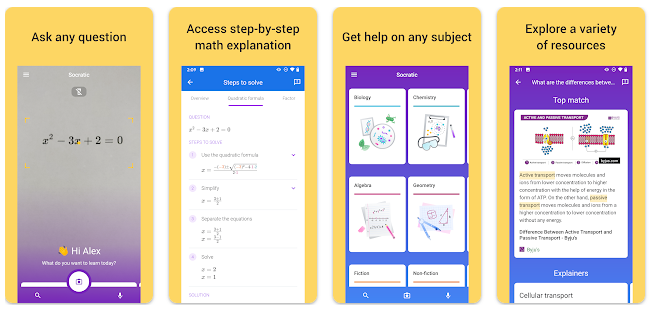Google also released an application to solve math problems with the ability to answer and explain tasks that are difficult to understand. The name of the app is Socratic. Apart from the formulas, it is also equipped with pictures, making it easier for you to understand it better.

Socratic is such an interesting application as it has various other subjects available, such as physics, biology, history, and literature. This application is clearly perfect for those who are doing online learning during a pandemic like this.

#### 6. Cymath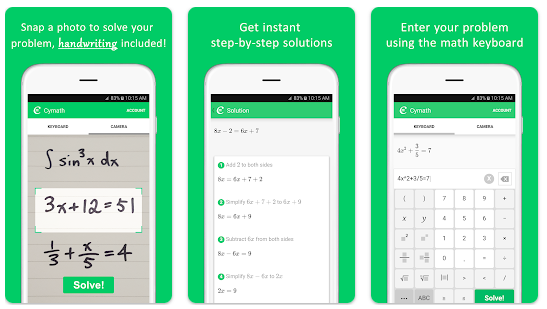From now on, you do not have to worry about doing math homework anymore, because there is a thing called Cymath. This one could be your friend to solve various types of lesson questions. To solve the math problems using this application, all that you have to do is to scan the questions on the camera of your phone and the application will provide answers to these questions. If you are worried about accuracy, please do not be because the application is supported by high technology so that the answers must be accurate.

#### 7. Microsoft Math Solver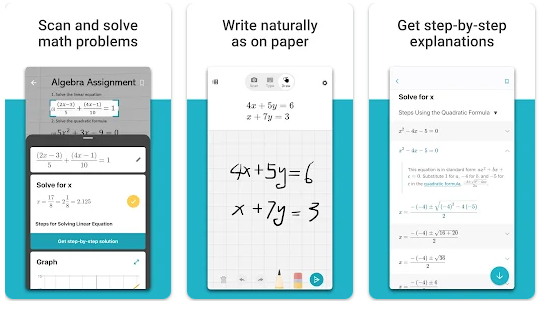To be able to get answers from Microsoft Math Solver, you will have to write questions in the application or take photos of the questions from the camera of your phone. After that, the application will provide reviews and answers quickly and everything will be easy to understand.

Microsoft Math Solver has the ability to work on topics ranging from addition, subtraction, division to arithmetic, algebra, trigonometry, calculus and statistics. It is perfect for all users.

#### 8. Symbolab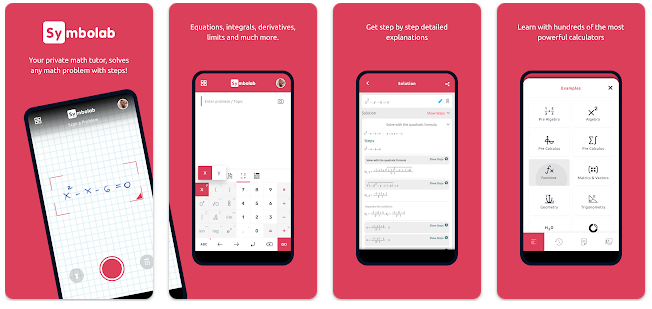Symbolab is the perfect solution for getting math answers. It is fast and easy to understand. Not only providing answers to questions, this application also presents the process of doing it in an attractive way. Besides, it can also be used as a calculator with a complex formula. The types of the calculator include a matrix calculator, integral calculator, derivative calculator, and so on.

#### 9. Gauthmath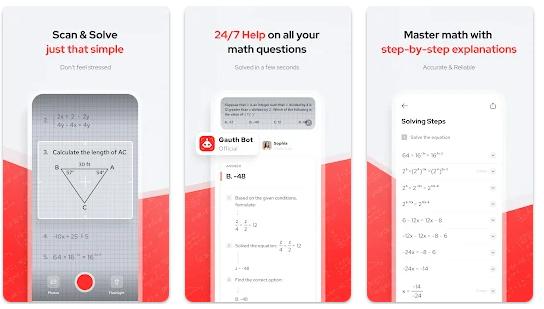Using Gathmath is like having your own personal tutor because it can help you with math homework. All that you have to do is to scan the questions on paper. After that, the tutor or teacher at Gauthmath will answer questions in the assignment. In order to make you understand, the tutor will provide an explanation of the answers to these questions. Go try this tutor feature now for free.

#### 10. Qanda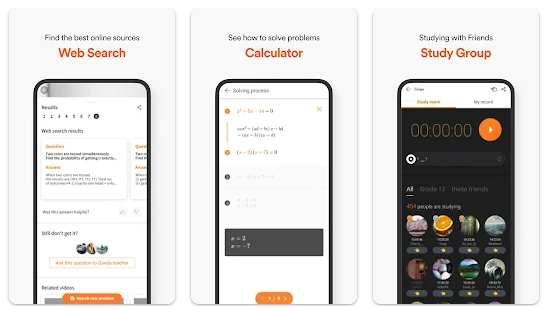The last application that you can consider to solve math is Qanda. This one will provide answers to math questions in detail so that you will be able to understand more after getting an explanation of the answers from the application, even though you initially do not understand certain formulas in math.

These are 10 math applications for Android to answer or work on math problems. Feel free to choose any application that you prefer and have fun doing your assignments.